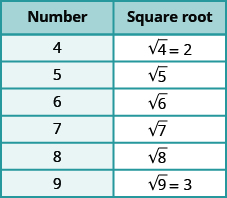## Estimating and Approximating Square Roots

### Learning Outcomes

• Estimate a square root that is not a perfect square
• Approximate square roots with a calculator

So far we have only worked with square roots of perfect squares. The square roots of other numbers are not whole numbers.We might conclude that the square roots of numbers between $4$ and $9$ will be between $2$ and $3$, and they will not be whole numbers. Based on the pattern in the table above, we could say that $\sqrt{5}$ is between $2$ and $3$. Using inequality symbols, we write

$2<\sqrt{5}<3$

### example

Estimate $\sqrt{60}$ between two consecutive whole numbers.

Solution
Think of the perfect squares closest to $60$. Make a small table of these perfect squares and their squares roots.$\text{Locate 60 between two consecutive perfect squares.}$ $49<60<64$ $\sqrt{60}\text{ is between their square roots.}$ $7<\sqrt{60}<8$

### try it

In the next video you will see more examples of how to estimate a square root between two consecutive whole numbers.

### Approximate Square Roots with a Calculator

There are mathematical methods to approximate square roots, but it is much more convenient to use a calculator to find square roots. Find the $\sqrt{\phantom{0}}$ or $\sqrt{x}$ key on your calculator. You will to use this key to approximate square roots. When you use your calculator to find the square root of a number that is not a perfect square, the answer that you see is not the exact number. It is an approximation, to the number of digits shown on your calculator’s display. The symbol for an approximation is $\approx$ and it is read approximately.

Suppose your calculator has a $\text{10-digit}$ display. Using it to find the square root of $5$ will give $2.236067977$. This is the approximate square root of $5$. When we report the answer, we should use the “approximately equal to” sign instead of an equal sign.

$\sqrt{5}\approx 2.236067978$

You will seldom use this many digits for applications in algebra. So, if you wanted to round $\sqrt{5}$ to two decimal places, you would write

$\sqrt{5}\approx 2.24$

How do we know these values are approximations and not the exact values? Look at what happens when we square them.

$\begin{array}{ccc}\hfill {2.236067978}^{2}& =& 5.000000002\hfill \\ \hfill {2.24}^{2}& =& 5.0176\hfill \end{array}$

The squares are close, but not exactly equal, to $5$.

### example

Round $\sqrt{17}$ to two decimal places using a calculator.

### try it

In the next video you will see more examples of ho to use a calculator to estimate the square root of a number.